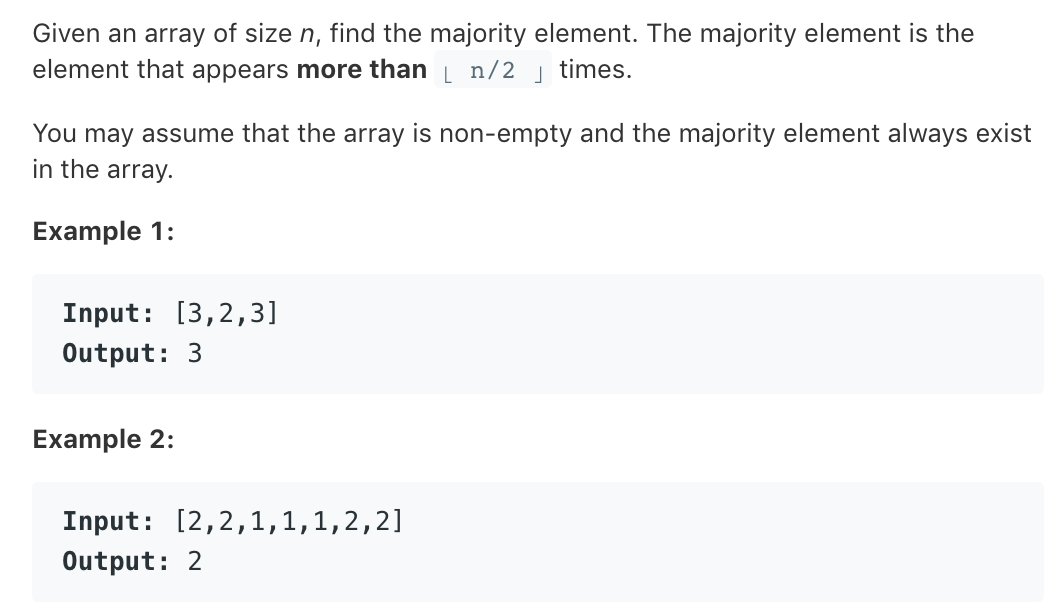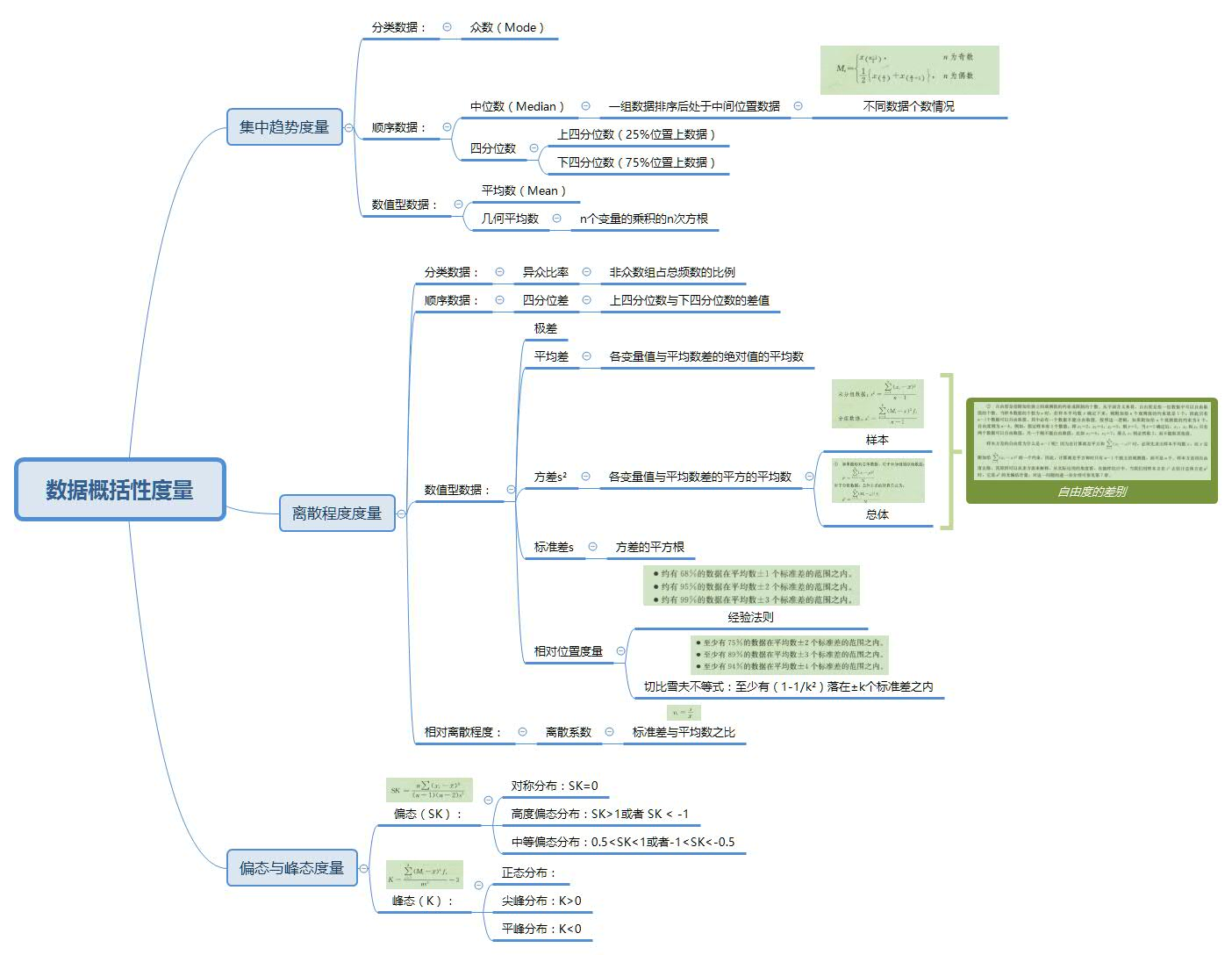• 本文实例讲述了python求众数问题的方法，是一个比较典型的应用。分享给大家供大家参考。具体如下： 问题描述: 多重集中重数最大的元素称为众数…就是一个可以有重复元素的集合,在这个集合中重复的次数最多的那个数就...
• ## 如何用Python求众数

千次阅读 2020-09-18 20:32:47
如何用Python求众数 在书里(参考文献)看到有这样的代码： max(set(A),key=A.count) 它可以返回列表A中的众数，现对其进行解释。 set 博客 python set() 用法.中提到：set() 函数用于创建一个无序不重复元素集，...
如何用Python求众数
在书里(参考文献)看到有这样的代码：
max(set(A),key=A.count)

它可以返回列表A中的众数，现对其进行解释。
set
博客 python set() 用法.中提到：set() 函数用于创建一个无序不重复元素集，删除重复数据。不过直接用max(A,key=A.count)，结果是一样的。
key
关于max()函数中的参数key，Python官方文档中写道：The key argument specifies a one-argument ordering function like that used for list.sort().
在论坛中搜索，一般情况下会用匿名函数lambda指定key，例如：
A=[3,5,5,5,1]
max_A=max(A,key=lambda x: x-1)
print(max_A)
#结果：5

但若是函数较为复杂，可以先def一个函数，而后将函数名传给key，例如：
def calculate(x):
return -(x-3)^2
A=[3,5,5,5,1]
max_A=max(A,key=calculate)
print(max_A)
#结果：3

因此猜想，方法也类同函数，因此可以将list.count（不需要写括号和实参）传给key，但list可以用于max的方法（要有返回值，且返回值可用于比较大小）只有.count()，故此猜想没有得到验证。
此外，list.sort()也有可选参数key，其官方文档中写到：for example, key=str.lower。此处同样是直接调用方法而没有写括号()，与本例相同。
参考文献
王磊,王晓东著.机器学习算法导论[M].北京:清华大学出版社,2019:182.


展开全文• python 求众数 LeetCode N0.169 class Solution(object): def majorityElement(self, nums): """ :type nums: List[int] :rtype: int """ count = 0 candidate = ...
python 求众数 LeetCode N0.169这道题有很多解法官方leetcode上面是六种，由于说的太过于详细，我都不好意思，再补充什么了。所以我就写了一点，没看答案之前的写法，和我觉得，需要掌握的写法吧。他写的很多代码很精简，值得学习。（ps，纳闷的是，即使我用的O（n）的复杂度，排名也很靠后哈哈哈哈哈）
class Solution(object):
def majorityElement(self, nums):
"""
:type nums: List[int]
:rtype: int
"""
#count = 0
#candidate = None

#for num in nums:
#   if count == 0:
#        candidate = num
#    count += (1 if num == candidate else -1)

#return candidate

count = 0
candidate = nums
for i in range(len(nums)):
if nums[i] == candidate:
count += 1
else:
count -= 1
if count == 0:
candidate =nums[i+1]
return candidate

class Solution(object):
def majorityElement(self, nums):
"""
:type nums: List[int]
:rtype: int
"""
import math
count = {}
if len(nums) %2 == 1:
n = len(nums)+1
else :
n = len(nums)
for i in nums:
count[i] = count.get(i,0) + 1

if count[i] >= n/2:
return i
for i in nums:
if count[i] >= n/2:
return i

class Solution:
def majorityElement(self, nums):
counts = collections.Counter(nums)
return max(counts.keys(), key=counts.get)

#作者：LeetCode
#链接：https://leetcode-cn.com/problems/majority-element/solution/qiu-zhong-shu-by-leetcode-2/
#来源：力扣（LeetCode）
#著作权归作者所有。商业转载请联系作者获得授权，非商业转载请注明出处。



展开全文LeetCode
• 数据概括性度量：python求众数、中位数、分位数、平均数 import pandas as pd #众数 #方法一 df = pd.read_excel("./data/例4.3.xlsx") data = df['月收入'] print('使用方法一,众数为：',data.mode().iloc) #...
数据概括性度量：python求众数、中位数、分位数、平均数import pandas as pd

#众数
#方法一
data = df['月收入']
print('使用方法一,众数为：',data.mode().iloc) #先取众数，iloc取行号（第一行）

#方法二
from collections import Counter
counts = Counter(data)
# most_common(1) ---> [(1),(2),(3),(4)] 列表里包含元组
# most_common(1) ---> (1) 将列表第一个元组取出来
# most_common(1) ---> 元组取第一个数据
print('使用方法二,众数为：',counts.most_common(1))

#方法三
from scipy import stats
mode_num = stats.mode(data)
print("使用方法三,众数为：%d, 众数的个数为：%d,"%(mode_num, mode_num))

#方法四
import numpy as np
# bin的数量比x中的最大值大1，每个bin给出了它的索引值在x中出现的次数
# 我们可以看到x中最大的数为7，因此bin的数量为8，那么它的索引值为0->7
# x = np.array([0, 1, 1, 3, 2, 1, 7])
# 索引0出现了1次，索引1出现了3次，索引2出现1次，索引3出现1次，索引4出现0次，索引5出现了0次...索引7出现1次
# np.bincount(x)
# 因此，输出结果为：array([1, 3, 1, 1, 0, 0, 0, 1])
counts = np.bincount(data)
print(counts)
print('使用方法四,众数为：',np.argmax(counts))

#中位数
#方法一
print('使用方法一,中位数为：' ,data.median())

#方法二
print('使用方法二,中位数为：' ,np.percentile(data, 50))

#方法三
# quantile的优点是与pandas中的groupby结合使用，可以分组之后取每个组的某分位数
print('使用方法三,中位数为：', data.quantile(0.50))

#分位数
#方法一
print('使用方法一,下四位数为：',np.percentile(data, 25))
print('使用方法一,上四位数为：',np.percentile(data, 75))

#方法二
print('下四分位数为：', data.quantile(0.25))
print('上四分位数为：', data.quantile(0.75))

#算数平均数
print('平均数为：',data.mean())
#几何平均数
import math
s = 1
for i in data:
s *= i
print('几何平均数为：',math.pow(s,1/len(data)))

#异众比率
modeCount = Counter(data).most_common(1)
totalCount = len(data)
radio = (totalCount - modeCount) / len(data)

#四分位差
# 法一
print('四分位差为：', np.percentile(data, 75) - np.percentile(data, 25))
# 法二
print('四分位差为：', data.quantile(0.75) - data.quantile(0.25))

# 极差
print('极差为：', data.max() - data.min())

# 平均差
meanData = data.mean()
s = 0
for i in data:
s += (abs(i - meanData))
print('平均差为：', s/len(data))

# 方差
print('方差为：', np.var(data))

# 标准差
print('标准差为：', np.std(data))

#偏度
print('偏度为：', stats.skew(data))

#峰度
print('峰度为：', stats.kurtosis(data))


[参考文档]https://mp.weixin.qq.com/s/VVi1DFsypcvXCpyJP_rD5Q
https://blog.csdn.net/qq_43315928/article/details/102151709
https://blog.csdn.net/xlinsist/article/details/51346523


展开全文数据分析
• 给定一个大小为 n 的数组，找到其中的众数众数是指在数组中出现次数大于 ⌊ n/2 ⌋ 的元素。 你可以假设数组是非空的，并且给定的数组总是存在众数。 示例 1: 输入: [3,2,3] 输出: 3 示例 2: 输入: ...
题目：

给定一个大小为 n 的数组，找到其中的众数。众数是指在数组中出现次数大于 ⌊ n/2 ⌋ 的元素。

你可以假设数组是非空的，并且给定的数组总是存在众数。

示例 1:

输入: [3,2,3]
输出: 3

示例 2:

输入: [2,2,1,1,1,2,2]
输出: 2

我的解答：

排序后众数就是数组正中间的数。

class Solution(object):
def majorityElement(self, nums):
"""
:type nums: List[int]
:rtype: int
"""
n = len(nums)
nums = sorted(nums)
if n % 2 == 0:
a = n / 2
return nums[a]
else:
a = (n - 1) / 2
return nums[a]
展开全文• [python]view plaincopy nums=[1,2,3,4] 均值和中位数均可以使用numpy库的方法： [python]view plaincopy importnumpyasnp #均值 np.mean(nums) #中位数 np.median(nums) 众...
• ## Python numpy求众数

万次阅读 2018-05-05 18:23:26
可以分别均值，中位数，但是却没有numpy.mode()求众数的函数。 那么如何求众数呢。当然是用 numpy.bincount。 可见bitcount返回数组值从0到最大值，各个值出现的个数。 要得到众数，直接找到返回...统计学
• 字符串找众数 train.loc[:,'Embarked'].describe().top 同理，将数字转为字符串找众数或用其他办法
• ## python计算众数

千次阅读 2020-10-07 16:44:13
计算众数 参数： list：列表类型，待分析数据 返回值： grade_mode: 列表类型，待分析数据的众数 ''' list_set = set(list) # 取list的集合，去除重复元素 frequency_dict = {} for i in list_set: # 遍历...
• 列表里出现次数最多的元素叫众数，使用python求众数目前没有直接的api，可以通过间接的方式求得众数，目前主要有以下几种方式。 暴力求解 # 暴力求解 import time login_list = [5, 8, 8, 5, 10, 9, 14, 16, 17, ...自然语言处理 机器学习 深度学习
• # what will i do:找到十个随机数之中的众数 # 统计数字的转换为 数字 和 出现次数 的格式 # 统计次数最多的 # 根据次数找下标 （先试试枚举） # 找到它 # def mn(xl,bl): #以字典形式 # pass # for i in xl: # bl[i...python
• 给定一个大小为 n 的数组，找到其中的众数众数是指在数组中出现次数大于 ⌊ n/2 ⌋ 的元素。 你可以假设数组是非空的，并且给定的数组总是存在众数。... 遍历字典出出现次数大于len(nums)//2 三、源码 # ...LeetCode
• 给定一个长度为 n 的数组，返回众数众数：是指数组中出现次数超过 n/2 次的元素。 假设数组非空，众数一定存在。 Example 1: Input: [3,2,3] Output: 3 Example 2: Input: [2,2,1,1,1,2,2] Output: 2 1：...
• 给定一个大小为 n 的数组，找到其中的众数众数是指在数组中出现次数大于 ⌊ n/2 ⌋ 的元素。 你可以假设数组是非空的，并且给定的数组总是存在众数。 示例 1: 输入: [3,2,3] 输出: 3 示例 2: 输入: [2,2,1,1,1,2,...
•中位数 峰度
• 平均数，中位数和众数是常用的表示数据水平的统计指标。当我们想要对比不同分组的数据的差异的时候，一般来说会用到平均数，但是平均数容易受到极端值的影响，这时候中位数和众数就是一个比较好的选择。 在pandas中...数据分析
• LeetCode刷题日记求众数Python代码 求众数 给定一个大小为 n 的数组，找到其中的众数众数是指在数组中出现次数大于 ⌊ n/2 ⌋的元素。 你可以假设数组是非空的，并且给定的数组总是存在众数。 示例 1: 输入: [3,2...LeetCode 数组...

# python求众数python 订阅